劳伦斯84 0 0# 如何用 JS 实现二叉堆

## 前言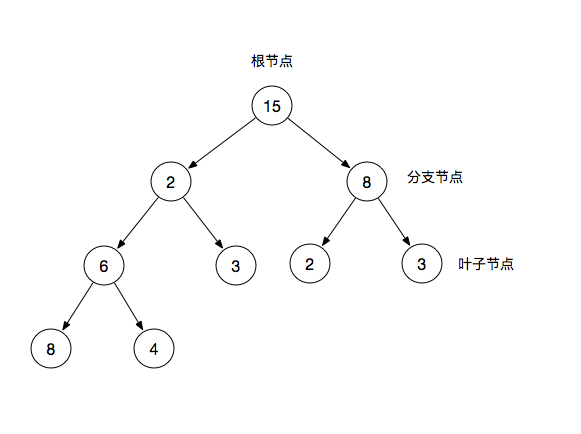## 二叉树与二叉堆的关系

### 二叉树特征

• 根节点：二叉树最顶层的节点
• 分支节点：除了根节点以外且拥有叶子节点
• 叶子节点：除了自身，没有其他子节点

### 二叉树分类

• 满二叉树：一棵深度为 k 且有 2 ^ k - 1个节点的二叉树称为满二叉树
• 完全二叉树：完全二叉树是指最后一层左边是满的，右边可能满也可能不满，然后其余层都是满的二叉树称为完全二叉树(满二叉树也是一种完全二叉树)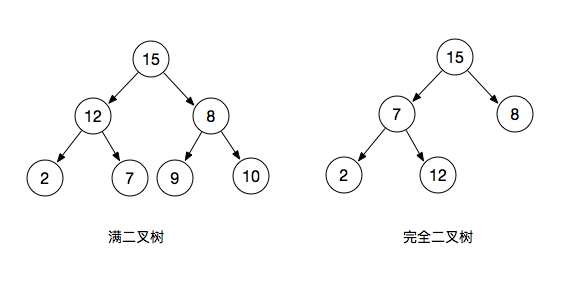### 二叉树结构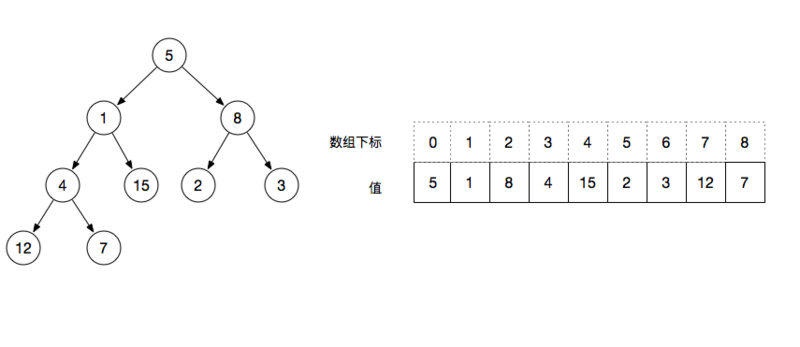• 二叉树左侧节点表达式 index * 2 + 1。例如：以根节点为例求左侧节点，根节点的下标为0，则左侧节点的序数是1 ，对应数组中的值为1
• 二叉树右侧节点表达式 index * 2 + 2。例如：以根节点为例求右侧节点，根节点的下标为0，则右侧节点的序数是2 ，对应数组中的值为 8
• 二叉树叶子节点表达式 序数 >= floor( N / 2 )都是叶子节点（N是数组的长度）。例如：floor( 9 / 2 ) = 4 ，则从下标 4 开始的值都为叶子节点

### 二叉堆特征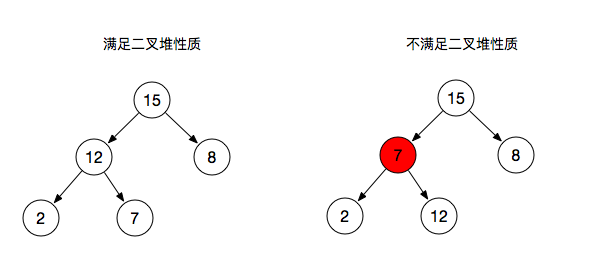• 图一：每个父节点大于子节点或等于子节点，满足二叉堆的性质
• 图二：其中有一个父节点小于子节点则不满足二叉堆性质

### 二叉堆分类

​ 二叉堆根据排序不同，可以分为最大堆和最小堆

• 最大堆：根节点的键值是所有堆节点键值中最大者，且每个父节点的值都比子节点的值大
• 最小堆：根节点的键值是所有堆节点键值中最小者，且每个父节点的值都比子节点的值小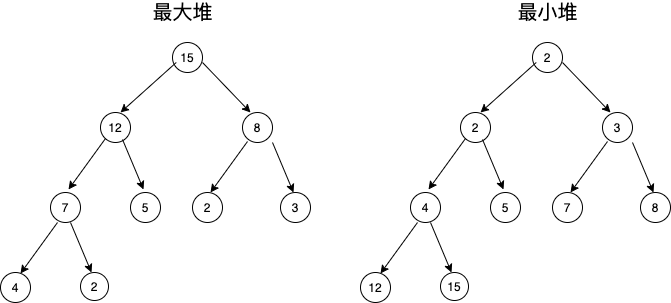)")

## 如何实现二叉堆

### 初始化二叉堆

class Heap{
constructor(arr){
this.data = [...arr];
this.size = this.data.length;
}
}

### 父子节点交换位置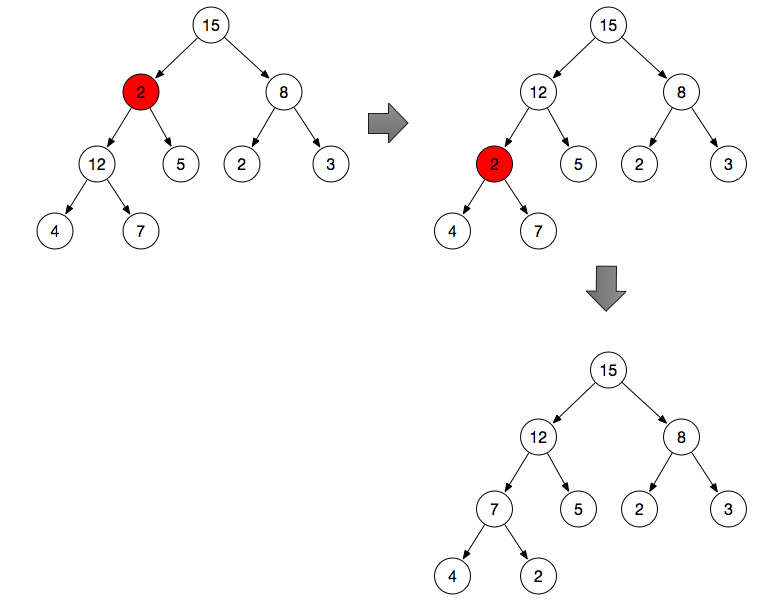1.调整分支节点 2 的位置（不满足最大堆性质）

2.获取父节点 2 的左右节点 ( 12 , 5 ) ，从 ( 2 , 15 , 5 ) 中进行比较

3.找出最大的节点与父节点进行交换，如果该节点本身为最大节点则停止操作

4.重复 step2 的操作，从 2 , 4 , 7 中找出最大值与 2 做交换（递归）

maxHeapify(i) {
let max = i;

if(i >= this.size){
return;
}
// 当前序号的左节点
const l = i * 2 + 1;
// 当前需要的右节点
const r = i * 2 + 2;

// 求当前节点与其左右节点三者中的最大值
if(l < this.size && this.data[l] > this.data[max]){
max = l;
}
if(r < this.size && this.data[r] > this.data[max]){
max = r;
}

// 最终max节点是其本身,则已经满足最大堆性质，停止操作
if(max === i) {
return;
}

// 父节点与最大值节点做交换
const t = this.data[i];
this.data[i] = this.data[max];
this.data[max] = t;

// 递归向下继续执行
return this.maxHeapify(max);
}

### 形成最大堆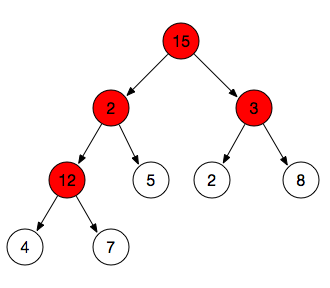1.找到所有分支节点 Math.floor( N / 2 )（不包括叶子节点）

2.将找到的子节点进行 maxHeapify 操作

rebuildHeap(){
// 叶子节点
const L = Math.floor(this.size / 2);
for(let i = L - 1; i >= 0; i--){
this.maxHeapify(i);
}
}

### 生成一个升序的数组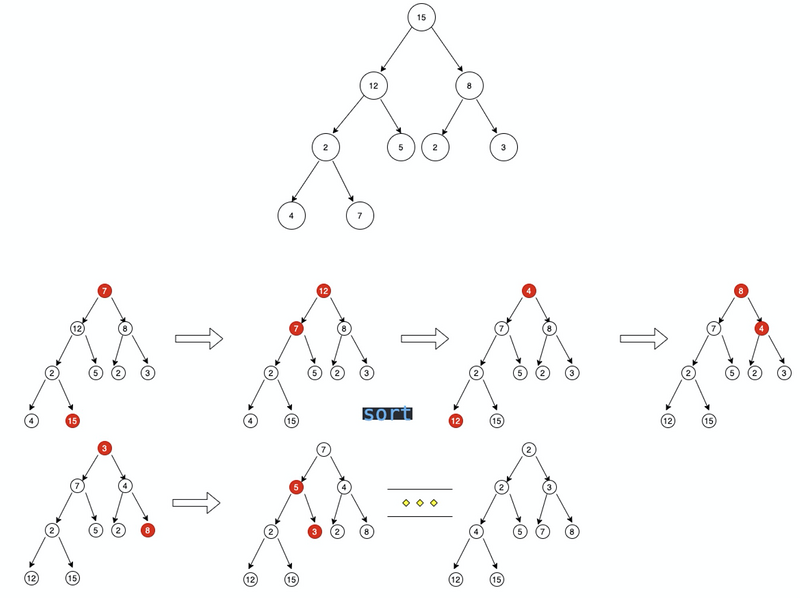1.swap 函数交换首位位置

2.将最后一个从堆中拿出相当于 size - 1

3.执行 maxHeapify 函数进行根节点比较找出最大值进行交换

4.最终 data 会变成一个升序的数组

sort() {
for(let i = this.size - 1; i > 0; i--){
swap(this.data, 0, i);
this.size--;
this.maxHeapify(0);
}
}

### 插入方法

Insert 函数作为插入节点函数，首先

1.往 data 结尾插入节点

2.因为节点追加，size + 1

3.因为一个父节点拥有 2 个子节点，我们可以根据这个性质通过 isHeap 函数获取第一个叶子节点，可以通过第一个叶子节点获取新插入的节点，然后进行 3 个值的对比，找出最大值，判断插入的节点。如果跟父节点相同则不进行重构（相等满足二叉堆性质），否则进行 rebuildHeap 重构堆

isHeap() {
const L = Math.floor(this.size / 2);
for (let i = L - 1; i >= 0; i--) {
const l = this.data[left(i)] || Number.MIN_SAFE_INTEGER;
const r = this.data[right(i)] || Number.MIN_SAFE_INTEGER;

const max = Math.max(this.data[i], l, r);

if (max !== this.data[i]) {
return false;
}
return true;
}
}
insert(key) {
this.data[this.size] = key;
this.size++
if (this.isHeap()) {
return;
}
this.rebuildHeap();
}

### 删除方法

delete 函数作为删除节点，首先

1.删除传入index的节点

2.因为节点删除，size - 1

3.重复上面插入节点的操作

delete(index) {
if (index >= this.size) {
return;
}
this.data.splice(index, 1);
this.size--;
if (this.isHeap()) {
return;
}
this.rebuildHeap();
}

### 完整代码

/**
* 最大堆
*/

function left(i) {
return (i * 2) + 1;
}

function right(i) {
return (i * 2) + 2;
}

function swap(A, i, j) {
const t = A[i];
A[i] = A[j];
A[j] = t;
}

class Heap {
constructor(arr) {
this.data = [...arr];
this.size = this.data.length;
this.rebuildHeap = this.rebuildHeap.bind(this);
this.isHeap = this.isHeap.bind(this);
this.sort = this.sort.bind(this);
this.insert = this.insert.bind(this);
this.delete = this.delete.bind(this);
this.maxHeapify = this.maxHeapify.bind(this);
}

/**
* 重构堆，形成最大堆
*/
rebuildHeap() {
const L = Math.floor(this.size / 2);
for (let i = L - 1; i >= 0; i--) {
this.maxHeapify(i);
}
}

isHeap() {
const L = Math.floor(this.size / 2);
for (let i = L - 1; i >= 0; i--) {
const l = this.data[left(i)] || Number.MIN_SAFE_INTEGER;
const r = this.data[right(i)] || Number.MIN_SAFE_INTEGER;

const max = Math.max(this.data[i], l, r);

if (max !== this.data[i]) {
return false;
}
return true;
}
}

sort() {
for (let i = this.size - 1; i > 0; i--) {
swap(this.data, 0, i);
this.size--;
this.maxHeapify(0);
}
}

insert(key) {
this.data[this.size++] = key;
if (this.isHeap()) {
return;
}
this.rebuildHeap();
}

delete(index) {
if (index >= this.size) {
return;
}
this.data.splice(index, 1);
this.size--;
if (this.isHeap()) {
return;
}
this.rebuildHeap();
}

/**
* 交换父子节点位置，符合最大堆特征
* @param {*} i
*/
maxHeapify(i) {
let max = i;

if (i >= this.size) {
return;
}

// 求左右节点中较大的序号
const l = left(i);
const r = right(i);
if (l < this.size && this.data[l] > this.data[max]) {
max = l;
}

if (r < this.size && this.data[r] > this.data[max]) {
max = r;
}

// 如果当前节点最大，已经是最大堆
if (max === i) {
return;
}

swap(this.data, i, max);

// 递归向下继续执行
return this.maxHeapify(max);
}
}

module.exports = Heap;

## 示例

const arr = [15, 12, 8, 2, 5, 2, 3, 4, 7];
const fun = new Heap(arr);
fun.rebuildHeap(); // 形成最大堆的结构
fun.sort();// 通过排序，生成一个升序的数组
console.log(fun.data) // [2, 2, 3, 4, 5, 7, 8, 12, 15]

## 招贤纳士